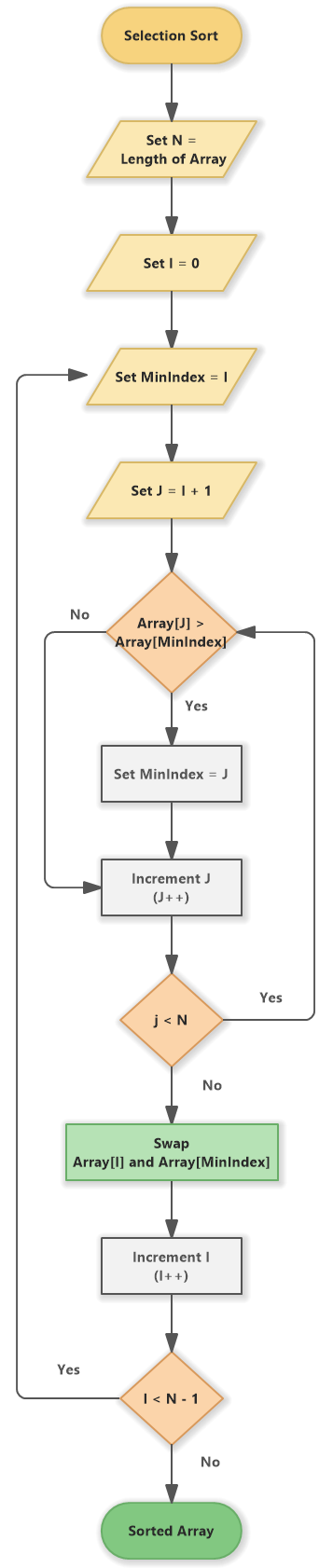# Selection Sort (Flowchart)

A flowchart for selection sort algorithm.

## Flowchart for Selection Sort

The flowchart shows the steps of selection sort algorithm.Selection Sort (Flowchart)

Selection Sort algorithm includes the following steps:

1. Set N = Length of Array

2. Set I = 0

3. Set MinIndex = I

4. Set J = I + 1

5. If Array[J] <= Array[MinIndex] continue with the step 8

6. Set MinIndex = J

7. Increment J (J++)

8. If j < N continue with the step 5

9. Swap Array[I] and Array[MinIndex]

10. Increment I (I++)

11. If I < N - 1 then continue with the step 2

12. The array is sorted.